Next: Monoids and Groups Up: Fundamentals Previous: Fundamentals

# Algebraic structures

We will attempt to give a brief explanation of the following concepts:

• N is a monoid
• Z is an integral domain
• Q is a field
• in the field R the order is complete
• the field C is algebraically complete

If you have been asked by a child to give them arithmetic problems, so they could show off their newly learned skills in addition and subtraction I'm sure that after a few problems such as: 2 + 3, 9 - 5, 10 + 2 and 6 - 4, you tried tossing them something a little more difficult: 4 - 7 only to be told ``That's not allowed.''

What you may not have realized is that you and the child did not just have different objects in mind (negative numbers) but entirely different algebraic systems. In other words a set of objects (they could be natural numbers, integers or reals) and a set of operations, or rules regarding how the numbers can be combined.

We will take a very informal tour of some algebraic systems, but before we define some of the terms, let us build a structure which will have some necessary properties for examples and counterexamples that will help us clarify some of the definitions.

We know that any number that is divided by six will either leave a remainder, or will be divided exactly (which is after all the remainder 0). Let us write any number by the remainder n it leaves after division by six, denoting it as [ n ]. This means that, 7, 55 and 1 will all be written , which we call the class to which they all belong: i.e.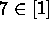,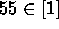, or, a bit more technically, they are all equivalent to 1 modulo 6. The complete set of class will contain six elements, and this is called partitioning numbers into equivalent classes because it separates (or partitions) all of our numbers into these classes, and any one number in a class is equivalent to any other in the same class.

One interesting thing we can do with these classes is to try to add or to multiply them. What can  +  mean? We can, rather naively try out what they mean in ``normal'' arithmetic:  +  = [1+3] = . So far so good, let us try a second example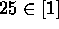and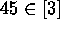, their sum is 70 which certainly belongs to . Here we see what we meant above by equivalence, 25 is equivalent to 1 as far as this addition is concerned. Of course this is just one example, but fortunately it can be proven that the sum of two classes is always the class of the sums.

Now this is the kind of thing we all do when we add hours for example, 7 (o' clock) plus 6 hours is 1 (o' clock), and all we are really doing is adding hours (modulo 12).

The neat part comes with multiplication, as we will see later on. But for now just remember, it can be proven that something like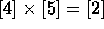will work: the product of two classes is the class of the product.

Now for some of the necessary terminology.Next: Monoids and Groups Up: Fundamentals Previous: Fundamentals

Alex Lopez-Ortiz
Mon Feb 23 16:26:48 EST 1998